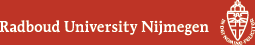## Geometry Seminar - Abstracts

### Talk

Tuesday 25 February 2020, 15:30-16:30 in HG02.802
Florian Zeiser (RU)
The Poisson cohomology of $$\mathrm{sl}_2(\mathbb{C})$$

### Abstract

To a Poisson manifold $$(M,\pi)$$ one can naturally associated a cohomology called Poisson cohomology. Although Poisson cohomology is important for questions such as linearization and deformations of poisson structures, it is in general quite difficult to compute it. In this talk we define Poisson cohomology. We look at its relation to the linearization problem and outline the general ideas behind the calculation of Poisson cohomology for $$\mathrm{sl}_2(\mathbb{C})$$. This is based on joint work with Ioan Marcut.

(Back to geometry seminar schedule)